Courses

# Test: Relation And Function (Competition Level) - 4

## 30 Questions MCQ Test Mathematics for JEE Mains | Test: Relation And Function (Competition Level) - 4

Description
This mock test of Test: Relation And Function (Competition Level) - 4 for JEE helps you for every JEE entrance exam. This contains 30 Multiple Choice Questions for JEE Test: Relation And Function (Competition Level) - 4 (mcq) to study with solutions a complete question bank. The solved questions answers in this Test: Relation And Function (Competition Level) - 4 quiz give you a good mix of easy questions and tough questions. JEE students definitely take this Test: Relation And Function (Competition Level) - 4 exercise for a better result in the exam. You can find other Test: Relation And Function (Competition Level) - 4 extra questions, long questions & short questions for JEE on EduRev as well by searching above.
QUESTION: 1

### The critical point of f (x) = |x- 1| is

Solution:

f (x) = |x- 1| is not diff at x = 1 critical point is at x = 1

QUESTION: 2

### IF f (x) = a log x + bx2 + x has extreme values at x = -1, x = 2 then a = ...., b = .....

Solution: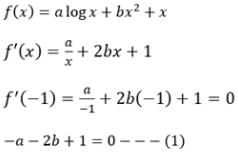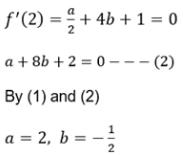QUESTION: 3

### If  x is real then the minimum value of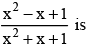Solution:

Min. value = f(1)
Max. Value = f(-1)

QUESTION: 4

The value of “a” for which the sum of the squares of the roots of the equation x2 - (a - 2) x - a - 1 = 0 assume the least value is

Solution: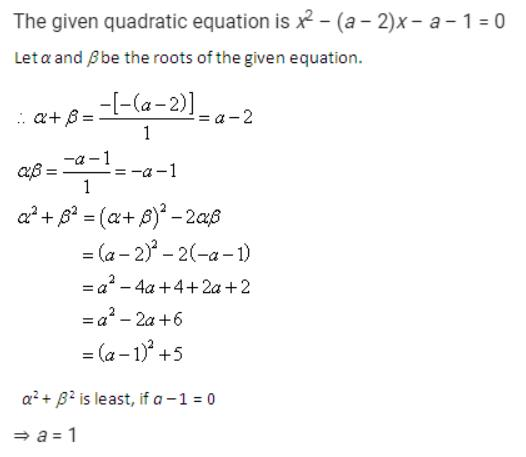QUESTION: 5

Greatest value of (1/x)x is

Solution:

Let y = (1/x)x
Taking ln both sides, we get ln y =x ln 1/x = x ln (x)-1
= -x ln x {Since,ln xa = a ln x  }
Now, differentiating both sides with respect to x we get,
1/y(dy/dx) = -1-ln x
⇒  (dy/dx) =(1/x)x [-1-ln x} = 0 {Condition for y to be maximum}

⇒ln x = -1  {Since, (1/x)x = 0}

⇒ x = e-1  {Converting logarithmic equation to exponential equation}

⇒ So,  y(max) = (e)1/e

QUESTION: 6

A wire of length 2 units is cut into two parts which are bent respectively to form a square of side = x unit and circle of radius = r units. If the sum of the area of the square and the circle so found is minimum then

Solution:

Given 4x + 2πr = 2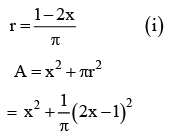For minimum value of area A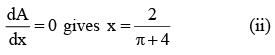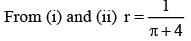x = 2r

QUESTION: 7

The function f (x) = 4x5 - 25x4 + 40x3 - 10 has

Solution:

f '(x) = 20x4 -100x3 + 120x2 f '(x) = 0
x = 2, 3 one maximum and one minimum

QUESTION: 8

The function x4 - 62x2 + ax + 9 attains its maximum value at x = 1, on the interval [0, 2] then value of ‘a’ is

Solution:

f (x) = x4 - 62x2 + ax + 9
f '(x) = 4x3 -124x + a
f '(1) = 0 at x =1 4 -124 + a = 0
x = 120

QUESTION: 9

The longest distance of the point (a, 0) from the curve 2x2 + y2- 2x is

Solution:

f (x) = (PA)2 = (x - a)2 +2x - 2x2
f '(x) = 0x = (1-a)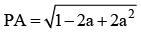QUESTION: 10

The point on the curve y = x2 which is nearest to (3, 0) is

Solution: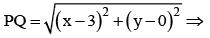Let Z=(x-3)2+y= x2-6x+9+x4
Z is minimum dz/dz = 2x-6+4x3 = 0
∴ x = 1 ∴ y = 1, (1,1)

QUESTION: 11

The difference between the greatest and least value of the function f (x) = sin 2x - x on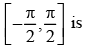Solution: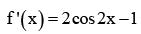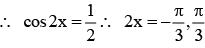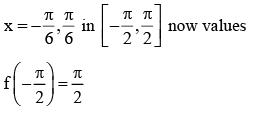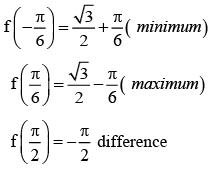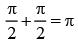QUESTION: 12

The sides of a rectangle of the greatest area which can be inscribed into an ellipse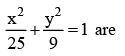Solution: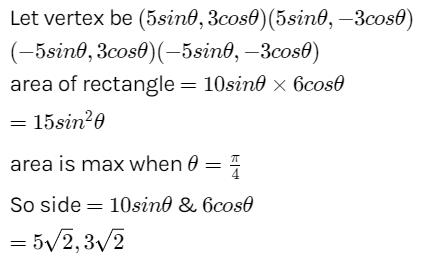QUESTION: 13

A window is in the shape of a rectangle surmounted by a semi circle. If the perimeter of the window is of fixed length „l‟ then the maximum area of the window is

Solution: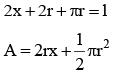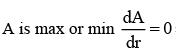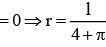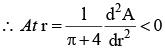QUESTION: 14

The sum of the hypotenuse and a side of a right triangle is constant. If the area of the triangle is maximum then the angle between the hypotenuse and the given side is

Solution:

z+x=k  z+zsinθ = k   z = k/1+sinθ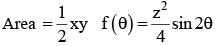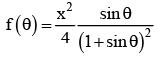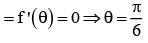QUESTION: 15

The number of linear functions which map from [-1,1] onto [0, 2] is

Solution:

Let f (x) = ax+ b be the required liner function.
If a > 0 then f is increa sin g, f(-1) = 0,f(1) = 2 ⇒ -a + b = 0,a +b = 2 ⇒ a = -1,b = 1
If a < 0 then f decrea sin g, f(-1) = 2,f (1) ⇒ = 0 -a + b = 2,a+b = 0 = ⇒ a = -1,b = 1
∴ The required linear function are f(x) = x+1,f(x) = -x+1.

QUESTION: 16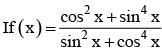for x ∈ R Then f (2002) =

Solution:

(cos2x + sin4x) - (sin2x + cos4x) = (cos2x - sin2x) - (cos4x - sin4x)
= cos2x - cos2x = 0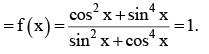QUESTION: 17

If f (0) = 0, f (1) = 1, f (2) = 2 and f (x) = f (x - 2) + f (x - 3) for x = 3, 4, 5,&.., then f(9) =

Solution:

Givenf (x) = f(x-2)+f(x-3)
∴ f(3) = f(1)+f(0) = 1 + 0 = 1,
f(4) = f(2)+f(1) = 2 + 1 = 3
f(5) = f(3)+f(2) = 1+ 2 = 3,f(6). f (4) + f(3) = 3+1 = 4,
f(7) = f(5)+f(4) = 3+3 = 6,f(8) = f(6)+f(5) = 4+3 =7,
f(9) = f(7)+f(6) = 6+4 = 10.

QUESTION: 18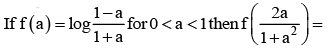Solution: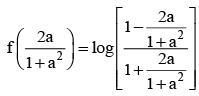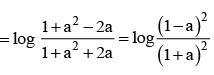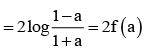QUESTION: 19

If f (x) = cos [π2] x + cos [- π2] x where [x] is the step function, then

Solution: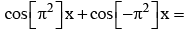cos 9x + cos (-10) x = cos 9x + cos10x

f (0) = cos0 + cos0 = 2,f (π/4) = cos9 π/4 + cos5π/2 = cos π/ 4 =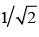f (π/2) = cos9π/2 + cos5π = 0 -1 = -l,f (π) = cos9π + cos10π = - l +1 = 0.

QUESTION: 20

If f (x) is a polynomial fuction such that f (x) f (1/x) = f (x)+ f (1/x) and f (2) = 33 then f (x) =

Solution:

f (x) f (1/x) = f (x) + f (1/x) ⇒ f(x) = xn +1.
f (2) = 33 ⇒ 2n +1= 33 ⇒ 2n = 32 = 25 ⇒ n = 5 ⇒ f (x) = x5 +1.

QUESTION: 21

If f (x) is a function such that f (x + y) = f (x) f (y) and f (3) = 125 then f (x) =

Solution:

f(x+y) + f(x) + f(y) ⇒ f(x) = ax.f(3)
= 125 ⇒ a3 = 125 ⇒ a = 5.
∴ f (x) = 5x

QUESTION: 22

If f : [2, ∞) → B defined by f (x) = x2 - 4x + 5is a bijection, then B =

Solution:

f (x) = x2 -4x +5 = (x -2)2 +1
x ∈ [2,∞) ⇒ x > 2 ⇒ x - 2 > 0 ⇒ (x - 2)2 +1 > 1 ⇒ B = [1,∞).

QUESTION: 23

The function f : C → C defined by f (x)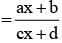for x ∈ C where bd ≠ 0 reduces to a constant function if

Solution: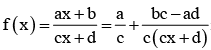is a constant function ⇒ bc ad 0 ⇒ ad bc.

QUESTION: 24

A function f : N → Z defined by f(n) = (n - 1)/2 when n is odd and f(n) = -n/2 when n is even, is

Solution:

Explanation : f(n-1)/2 ; n is odd        -n/2 ; n is even

f(1) = 0                f(3) = 1

f(2) = -1               f(4) = -2

f(5) = 2                f(6) = -3

f(n) + f(n+1) = -1    n is odd

if = 0     n is even

Range of f(n) = Z

f(n) is one-one, therefore f(n1) = f(n2)

(n1-1)/2 = -n2/2

=> 1 < (n1 + n2)/2 is not equal to 1/2

Therefore f(n) is onto.

QUESTION: 25

A = {x : |x| < 1|} and f : A → A such that f (x) = x |x|, then

Solution: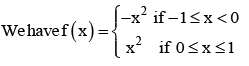Graph of y = f (x) is given in the figure.
∴ f is bijective.

QUESTION: 26

The domain of the function f = {(1, 3), (3, 5), (2, 6)} is

Solution:

Domain = {1, 3, 2}

QUESTION: 27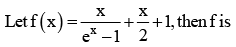Solution: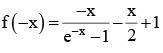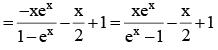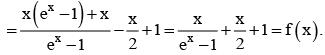∴ f is an even function.

QUESTION: 28

The range of f(x)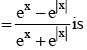Solution:

If x > 0 then f x = 0. If x < 0 then f (x) = tanh x. If f (x) = -1then
ex - e|x| = - ex - e|x| ⇒ex = 0 ⇒ x ∉ R.
Range does not contain -1.
∴ Range = (-1, 0].

QUESTION: 29

The domain of log (x - 3) (5- x) is

Solution:

log (x - 3) (5 - x) exists ⇒ (x - 3) (5 - x) > 0
⇒ (x -3)(x -5) < 0 ⇒ 3< x < 5
∴ Domain = (3, 5).

QUESTION: 30

The domain of log10 (x3 - x) is

Solution:

log10 (x3 - x)is defmed ⇒ x3 - x > 0 ⇒ x(x2 - 1) > 0 ⇒ (x +1)x(x - 1) > 0
x < -1 ⇒ (x+1)x(x-1) < 0; -1 < x < 0 ⇒ (x+1)x(x-1) > 0
0 < x < 1 ⇒ (x+1)x(x-1) < 0; x > 1 ⇒ (x+1)x(x-1) > 0.
∴ Domain = (-1,0)∪(1, ∞)